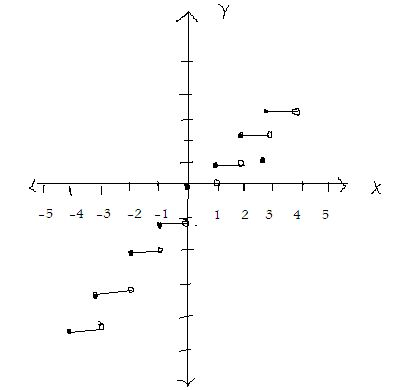kwizNET Subscribers, please login to turn off the Ads!
 Email us to get an instant 20% discount on highly effective K-12 Math & English kwizNET Programs!

#### Online Quiz (WorksheetABCD)

Questions Per Quiz = 2 4 6 8 10

### High School Mathematics - 24.2 Step Function or the Greatest Integer Function

 The figure below shows the graph of the function s, where x € R and s(x) = {1 if x > 0; 0 if x = 0; -1 if x < 0.This function is called step function. The step function G , partially graphed below maps each real number to x into the greatest integer that is less than or equal to x. [] means step integer function.For instance, G maps 2 into 2 because the greatest integer less than or equal to 2 is 2 itself. But G also maps 2 1/2 into 2, since the greatest integer less than or equal to 2 1/2 is 2. To denote this, we use the notation G = x -> [x] or we write G(x) = [x], where the symbol [x] is read the "the greatest integer that is less than or equal to x." Thus,  = 2, [2 1/2]= 2, [2.69] = 2, but [-2 1/2] = -3,  = 0, [1 1/3] = 1, [-1/5] = -1, [2.99] = 2, [-1] = -1, [4 3/4] = 4, [-3 1/10] = -4, [-5.1] = -6 Definition: Let x € R, A function f(x) = [x] where f(x) = n (an integer) such that n<=x< n+1 is called a step or greatest integer function. Thus for all x such that 0<=x<1, f(x) = 0, 1<=x<2, f(x) = 1 2< = x<3, f(x) = 2 ............ ........... .............. ........... -1<=x<0 f(x) = -1 -2<=x<-1 f(x) = -2 ............. ............. ............. .............. Domain of f = R, Range of f = set of integers Example: Draw the graph of the following function y = [x] in -2<=x<4 Solution: y = [x]. Here the value of y is the integer value of x. Some points of the graph are When x is -2<=x<1, y is -2 When x is -1<=x<1, y is -1 When x is 0<=x<1, y is 0 When x is 1<=x<1, y is 1 When x is 2<=x<1, y is 2 When x is 3<=x<1, y is 3 i.e f(x) = { -2 when x € [-2,-1] -1 when x € [-1, -0] 0 when x € [0,1] 1 when x € [1,2] and so on. The graph is as shown below. Clearly the function has jumps at the points (-1,-2), (0,-1), (1,0), (2,1) etc. In other words, the given function is discontinuous at each integral value of x. Directions: Answer the following.
 Q 1: Draw the graph of the function f: R-> R defined by f(x) = |x - 2|Answer: Q 2: Draw the graph of the function f: R-> R defined by f(x) = 2x+3Answer: Q 3: Draw the graph of the function f: R-> R defined by f(x) = 2x+3Answer: Q 4: Draw the graph of the function f: R-> R defined by f(x) = |x|Answer: Question 5: This question is available to subscribers only! Question 6: This question is available to subscribers only!

#### Subscription to kwizNET Learning System offers the following benefits:

• Unrestricted access to grade appropriate lessons, quizzes, & printable worksheets
• Instant scoring of online quizzes
• Progress tracking and award certificates to keep your student motivated
• Unlimited practice with auto-generated 'WIZ MATH' quizzes
• Child-friendly website with no advertisements
• Choice of Math, English, Science, & Social Studies Curriculums
• Excellent value for K-12 and ACT, SAT, & TOEFL Test Preparation
• Get discount offers by sending an email to discounts@kwiznet.com

 Quiz Timer